Chain-Base Index Numbers, Business Mathematics and Statistics

# Chain-Base Index Numbers, Business Mathematics and Statistics - Business Mathematics and Statistics - B Com

CHAIN INDEX NUMBERS

In the fixed base method which is discussed so far the base remains the same & does not change whole throughout the series. But with the passage of time some items may have been included in the series & other ones might have been deleted, & hence it becomes difficult to compare the result of present conditions with those of the old remote period. Hence the fixed base method does not suit when the conditions change. In such a case the changing base period may be more suitable. Under this method the figures for each year are first expressed as a percentage of the preceding year (called link relatives) then they are chained together by successive multiplication to form a chain index.

STEPS IN CONSTRUCTION OF CHAIN INDEX

(1) The figures are to be expressed as the percentage of the preceding year to get link relatives.
Link Relatives of current year =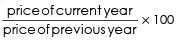(2) Chain index is obtained by the formula :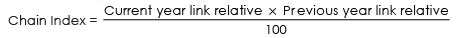Example 8: From the following data find the index numbers by taking (1) 2005 as base (ii) by chain base method.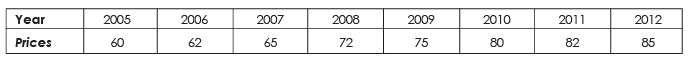Solution
Table :
Construction of Index Number taking 2005 as base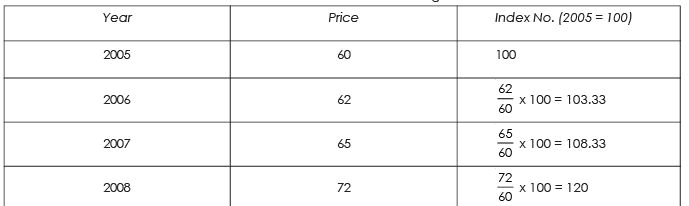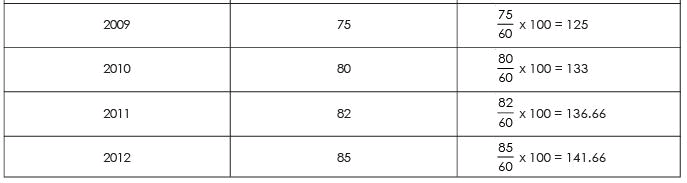This means that from 2005 to 2006 there is an increase of 3.33% (103.33 - 100) from 2005 to 2007 there is an increase of 8.33% (108.33 - 100) increase from 2005 to 2008 there is an increase of 20% (120 - 100) & so on ..........

Table : Calculation of Chain Base Index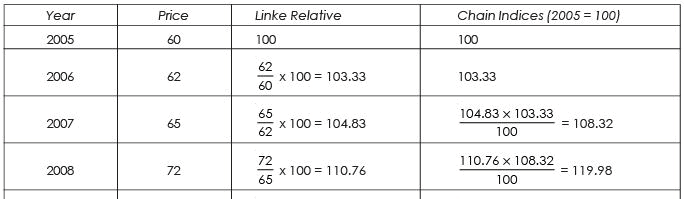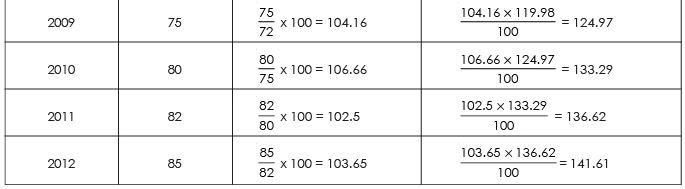Note: The results obtained by the fixed base & chain base are almost similar. The difference is only due to approximation. Infact the chain base index numbers are always equal to fixed base index numbers if there is only one series.

Example 9 :
From the following data compute chain base index number & fixed base index number.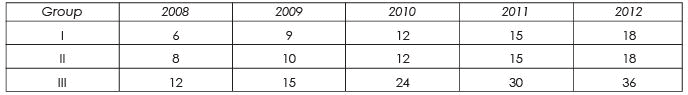Solution :
Table :
Computation of Chain Base Index Number Calculation of Link Relatives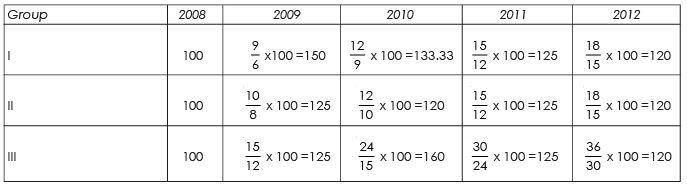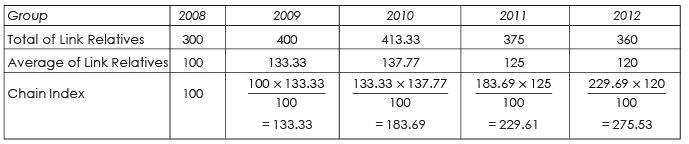Table : Computation of Fixed base Index No.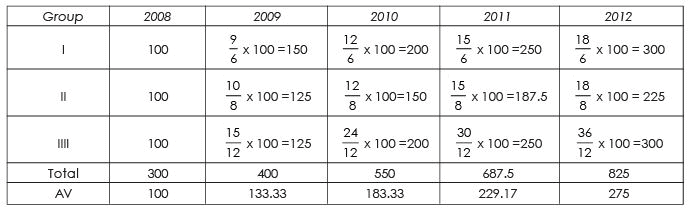Note : The two series of index numbers obtained by fixed base & chain base method are different except in the first two years. This would always be so in case of more than one series.

Example 10 : The price index & quantity index of a commodity were 120 & 110 respectively in 2012 with base 2011. Find its value index number in 2012 with base 2011.
Solution :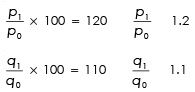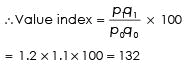The document Chain-Base Index Numbers, Business Mathematics and Statistics | Business Mathematics and Statistics - B Com is a part of the B Com Course Business Mathematics and Statistics.
All you need of B Com at this link: B Com

115 videos|142 docs

## FAQs on Chain-Base Index Numbers, Business Mathematics and Statistics - Business Mathematics and Statistics - B Com

 1. What are Chain-Base Index Numbers?Ans. Chain-Base Index Numbers are a type of index number that measure the changes in the value or quantity of a variable relative to a base period. They are computed by linking together the index numbers of consecutive time periods, using the value of the previous period as the new base for the next period.
 2. What is the purpose of using Chain-Base Index Numbers?Ans. The purpose of using Chain-Base Index Numbers is to provide a more accurate measure of changes in the value or quantity of a variable over time. By using a base period that changes with each time period, Chain-Base Index Numbers take into account any shifts in the underlying data and can better reflect the current state of the economy or market.
 3. How are Chain-Base Index Numbers calculated?Ans. Chain-Base Index Numbers are calculated by linking together the index numbers of consecutive time periods. To do this, the index number for the current period is divided by the index number for the previous period, and then multiplied by 100. This gives the percentage change in the value or quantity of the variable between the two periods.
 4. What are some advantages of using Chain-Base Index Numbers?Ans. Some advantages of using Chain-Base Index Numbers include: - They provide a more accurate measure of changes in the value or quantity of a variable over time. - They take into account any shifts in the underlying data and can better reflect the current state of the economy or market. - They are less affected by the choice of base period than fixed-base index numbers. - They can be updated easily and frequently, allowing for more timely and accurate analysis.
 5. What are some limitations of using Chain-Base Index Numbers?Ans. Some limitations of using Chain-Base Index Numbers include: - They can be more complex to calculate than other types of index numbers. - They may be more sensitive to short-term changes in the data, which can lead to greater volatility and less stability over time. - They may not provide as clear of a picture of long-term trends as fixed-base index numbers, which may be better suited for certain types of analysis.

115 videos|142 docsExplore Courses for B Com examSignup to see your scores go up within 7 days! Learn & Practice with 1000+ FREE Notes, Videos & Tests.
10M+ students study on EduRev
Track your progress, build streaks, highlight & save important lessons and more!
Related Searches

,

,

,

,

,

,

,

,

,

,

,

,

,

,

,

,

,

,

,

,

,

,

,

,

;